# FPGA-Based Beamforming in Simulink: Code Generation

This example shows the second half of a workflow to generate HDL code for a beamforming algorithm and verify that the generated code is functionally correct.

The first part of the example, FPGA-Based Beamforming in Simulink: Algorithm Design, shows how to develop an algorithm in Simulink® suitable for implementation on hardware, such as a field programmable gate array (FPGA), and how to compare the output of the fixed-point implementation model to that of the corresponding floating-point behavioral model.

This example uses HDL Coder™ to generate HDL code from the Simulink model developed in the first part and verifies the HDL code using HDL Verifier™. HDL Verifier is used to generate a cosimulation test bench model to verify the behavior of the generated HDL code. The test bench uses ModelSim® for cosimulation to verify the automatically generated HDL code.

The Phased Array System Toolbox™ Simulink blocks model operations on frame-based, floating-point data and provides the behavioral reference model. The example uses this behavioral model to verify the results of the implementation of the algorithm for hardware and the generated HDL code.

HDL Coder generates portable synthesizable Verilog® and VHDL® code for over 300 Simulink blocks that support HDL code generation. Those Simulink blocks operate on serial data using fixed-point arithmetic with delays included to enable pipelining by the synthesis tool.

HDL Verifier helps you test and verify Verilog and VHDL designs for FPGAs, ASICs, and SoCs. This example verifies HDL generated from a Simulink model against a test bench running in Simulink using cosimulation with an HDL simulator.

### Implementation Model

This tutorial assumes that you have a Simulink model that contains a subsystem with a beamforming algorithm designed using Simulink blocks that use fixed-point arithmetic and support HDL code generation. The FPGA-Based Beamforming in Simulink: Algorithm Design example shows how to create such a model.

Alternatively, if you start with a new model, you can run `hdlsetup` (HDL Coder) to configure the Simulink model for HDL code generation. To configure the Simulink model for test bench creation, open the Model Settings, select Test Bench under HDL Code Generation in the left panel, and check HDL test bench and Cosimulation model in the Test Bench Generation Output properties group.

### Comparing Results of Implementation Model to Behavioral Model

Run the model created in the FPGA-Based Beamforming in Simulink: Algorithm Design example to display the results. You can run the Simulink model by clicking the Play button or calling the sim command from the MATLAB® command line. Use the Time Scope blocks to compare the output frames visually.

```modelname = 'SimulinkBeamformingHDLWorkflowExample'; open_system(modelname); % Ensure model is visible and not obstructed by scopes. set(allchild(0),'Visible','off'); sim(modelname); ```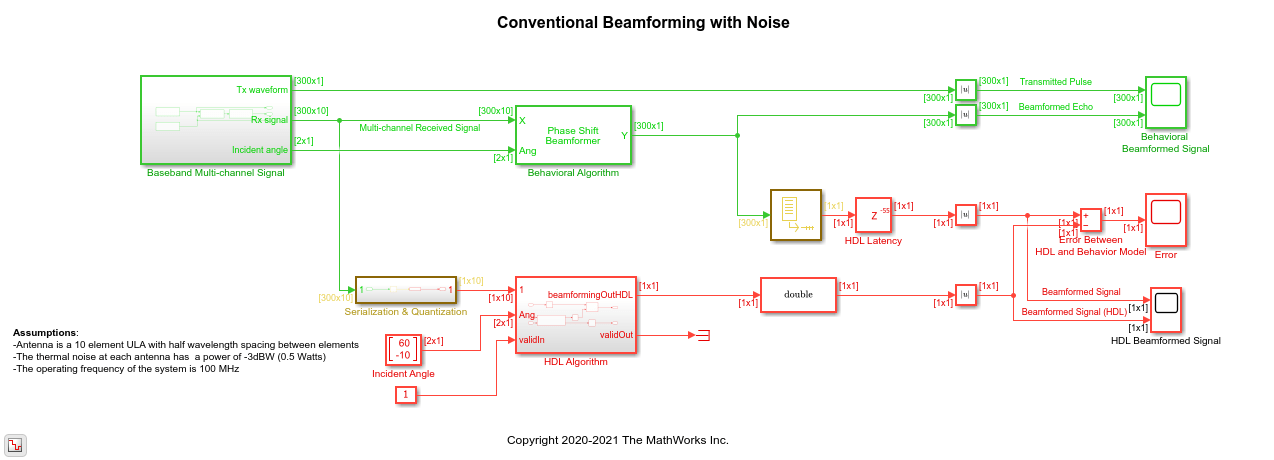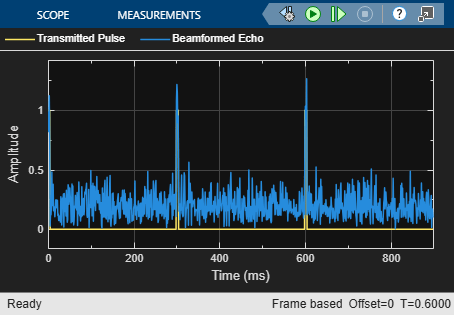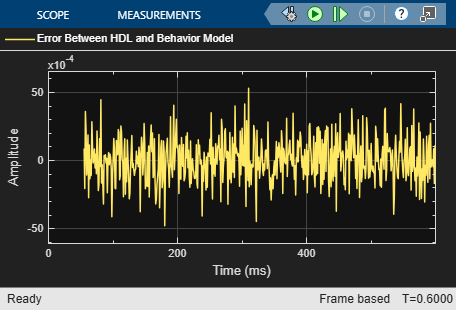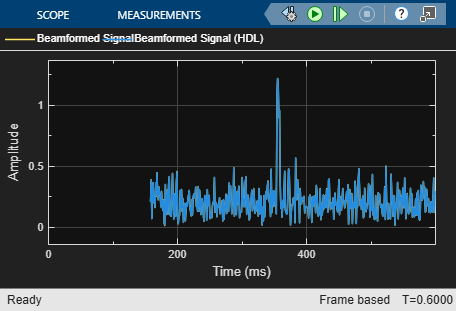### Model Settings

Once you verify that your fixed-point implementation model produces the same results as your floating-point behavioral model, you can generate HDL code and test bench. First, set the HDL Code Generation parameters in the Simulink Configuration Parameters dialog. For this example, set the following parameters in Model Settings under HDL Code Generation:

• Target: Xilinx Vivado synthesis tool; Virtex7 family; Device xc7vx485t; package ffg1761, speed -1; and target frequency of 300 MHz.

• Optimization: Uncheck all optimizations except Balance delays.

• Global Settings: Set the Reset type to Asynchronous.

• Test Bench: Select HDL test bench and Cosimulation model.

The reason the optimizations are disabled in this model is because some blocks used in our implementation are already HDL-optimized blocks, which could conflict with the HDL Coder optimizations.

### HDL Code Generation and Test Bench Creation

Next, use HDL Coder to generate HDL code for the `HDL Algorithm` subsystem. For an example of how to generate HDL code, see Generate HDL Code from Simulink Model (HDL Coder).

Use these commands to generate HDL code and test bench.

```makehdl([modelname '/HDL Algorithm']); % Generate HDL code makehdltb([modelname '/HDL Algorithm']); % Generate Cosimulation test bench ```

When you call the `makehdl` command, the MATLAB command window shows the amount of delay added during the automatic code generation process. In this case, 24 delays are added which results in an extra delay of 24*1ms = 24ms. The final output is expected to be delayed this additional amount, for a total delay of 79ms.

Because of this extra delay added during code generation, the output of the floating-point behavioral model needs to be delay balanced by adding 24 delays to the original 55. This delay aligns the output of the behavioral model with the implementation model and the cosimulation output.

The process of generating the HDL code and test bench creates a new Simulink model in the working folder, named `gm_<modelname>_mq`. The model contains a ModelSim Cosimulation block.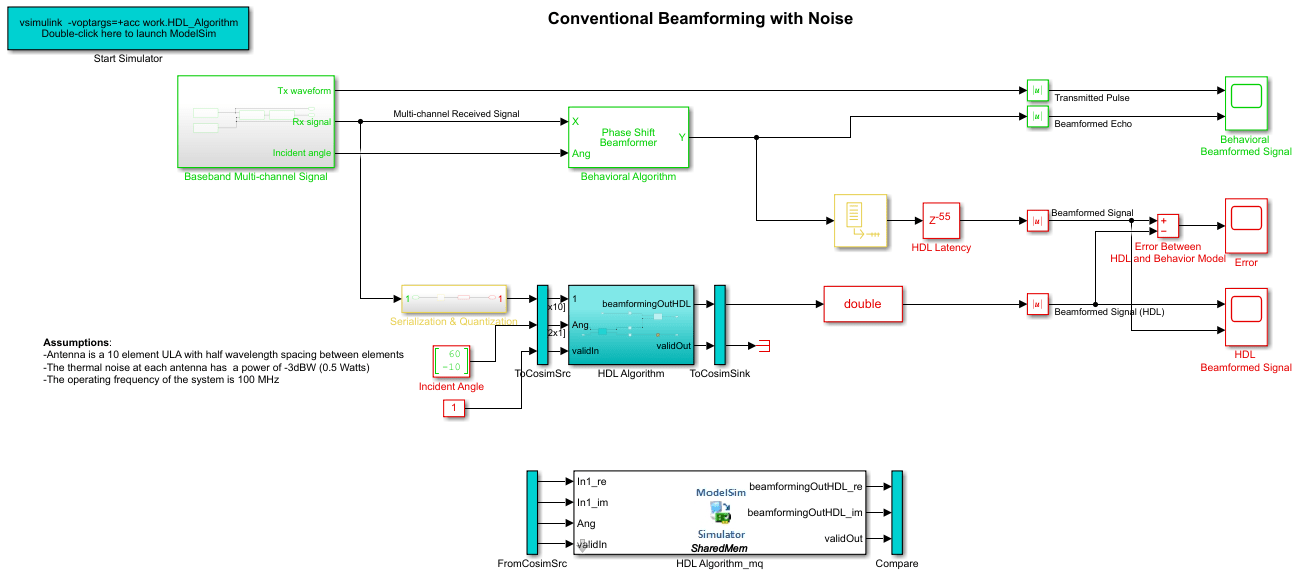Use these commands to open the test bench model.

```modelname = ['gm_',modelname,'_mq']; open_system(modelname); ```

In this new model, change the delay setting in the HDL Latency block to 79 to account for the 24 delays added by the code generation process. Using a delay of 79 ensures that the behavioral model output is time-aligned with the output of the implementation and cosimulation output.

### HDL Code Verification via Cosimulation

Before opening ModelSim make sure that the command to start ModelSim, `vsim`, is on the path of your machine.

To run the cosimulation model, double-click the blue rectangular box in the upper-left corner of the Simulink test model to launch ModelSim.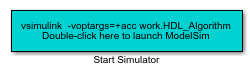Run the Simulink test bench model to display the simulation results. You can run the Simulink model by clicking the Play button or calling the sim command on the MATLAB command line. The test bench model includes Time Scope blocks to compare the output of the cosimulation performed with ModelSim with the output of the HDL subsystem in Simulink.

If you have ModelSim on your path, use this command to run the test bench.

```sim(modelname); ```

After starting ModelSim, running the Simulink test bench model will populate Questa Sim with the HDL model's waveforms and Time Scopes in Simulink. The figures show examples of the results in Questa Sim and Simulink scopes.

NOTE: You must restart Questa Sim each time you want to run the Simulink simulation. To restart, call `restart` at the Questa Sim command line. Alternatively, you can quit Questa Sim and re-launch it by double-clicking the blue box in the upper-left corner of the Simulink test bench model.The Simulink scope shows both the cosimulation and HDL model (DUT) producing a 79ms delayed version of the original signal produced by the behavioral model, as expected, with no difference between the two waveforms. The 79ms delay is due to the original 55ms delay added in the `HDL Algorithm` subsystem to enable pipelining by the synthesis tool and an additional 24ms delay due to the delay balancing added by HDL code generation. The additional 24 delays are reported during the code generation step.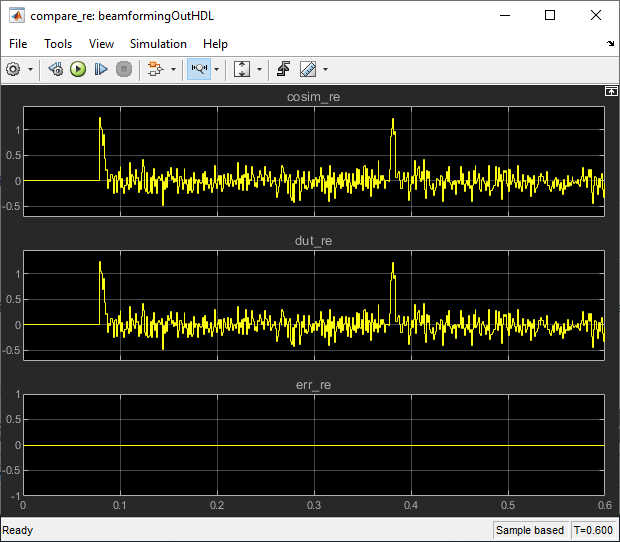The Simulink scopes comparing the results of the cosimulation can be found in test bench model inside the `Compare` subsystem, which is at the output of the `HDL Algorithm_mq` subsystem.

Use this command to open the subsystem with the scopes.

```open_system([modelname,'/Compare/Assert_beamformingOutHDL']) ```

### Summary

This example is the second of a two-part tutorial series on how to automatically generate HDL code for a fixed-point, sample-based beamforming algorithm and verify the generated code in Simulink. The first part of the tutorial, FPGA-Based Beamforming in Simulink: Algorithm Design, shows how to develop an algorithm in Simulink suitable for implementation on an FPGA. This example showed how to set up a model to generate the HDL code and a cosimulation test bench for a Simulink subsystem created with blocks that support HDL code generation. It showed how to set up and launch ModelSim to cosimulate the HDL code and compare its output to the output generated by the HDL implementation model.